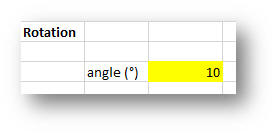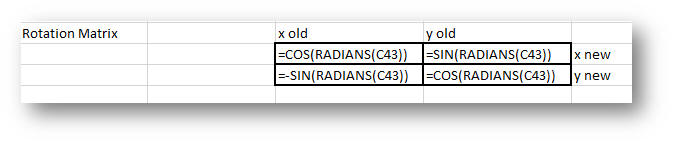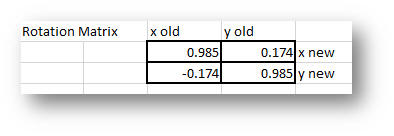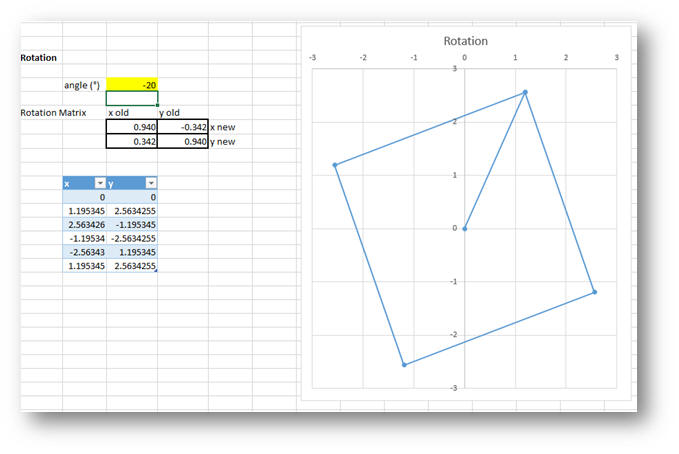# Excel Fun—Build 3D graphics from a spreadsheet

Today’s post was written by Carl Kadie, Ph.D., a research developer in Microsoft Research.

### How Excel helps to understand 3D graphics

From video games to movie special effects, 3D graphics drives today’s entertainment. But how do 3D graphics work? How does the computer know where on the screen to put the little dots of color? Motivated by simple curiosity, I wanted to understand this. And for me, to understand means to make an Excel spreadsheet. This article should interest you, too, if you are curious about 3D graphics. It also serves as an example of how Excel can make something as complex as 3D graphics as simple as a few worksheet cells connected by multiplication and addition.

In the end, I didn’t replicate Halo, but I did get lovely cube that I can control by changing a few numbers in Excel. For example, one cell tells how many degrees to rotate the cube. When I change the value in that cell from 20 degrees (figure on left) to 5 degrees (figure on right), the cube changes:

You can try it, too. In the embedded spreadsheet below, change the value in yellow, press Enter and to see how the figure changes. Alternatively, download the spreadsheet and play with it in Excel by changing the values in yellow.

### The key to the puzzle—rotate a square

Inspired by Einstein’s quote, “Everything should be made as simple as possible, but not simpler,” I set a goal to create and rotate a 2D square. But how in Excel do you even draw a square (let alone rotate it)? We don’t typically think of Excel as a drawing program. Its XY (scatter) chart, however, you can take a list of points (each with an x- and y-coordinate) and connect the dots. So, I listed a square’s center point and its four corners (figure on left): and Excel gave me this (figure on right):

Now that I had my square, I needed to figure out how to rotate it. Happily, a Wikipedia article told me exactly what to do. Sadly, the article is written in the language of matrix mathematics, so I had to convert the instructions into a 2 x 2 table and then apply the multiplication and addition. Here is what I did:

Created a place to input the number of degrees we want the square to rotate. In the spreadsheet, this is cell C43 and I highlighted it in yellow and gave it value of 10.Next, I created a 2 x 2 “rotation matrix” with formulas, from the Wikipedia article, which used only cell C43.Given an input of 10 degrees, the formulas result in these numbers:The first row says that to create a new x-coordinate for a point, take .985 of its old x-coordinate and .174 of its old y-coordinate. Similarly, the second row says that to create a new y-coordinate for a point we take -.174 time its old x-coordinate plus .985 of its old y-coordinate.

Doing this multiplication and addition for each of the points resulted in a second table of points. (Notice the first point was <0,0> before and is <0,0> after. I discovered, unsurprisingly, that rotating something at the center of the universe doesn’t change its position.) When I plotted the new table of points, I saw my rotation!

Changing the input from 10 degrees to –20 degrees, shifted the cube:Again, you can try this in following embedded spreadsheet. Simply, change the value in yellow, press Enter and see how the figure changes.

After I got 2D rotation working, the rest followed the same pattern and fell into place. When you open the spreadsheet, you’ll see the 2D rotation, then the 2D translation and finally 2D scaling. Tabbing to the second worksheet, you’ll find the 3D cube, which starts with a cube (defined with one center point and 16 corner points), three rotations (because in 3D there are three ways to rotate), translation, scaling and then a couple of ways to do perspective.

### Beyond graphic rotation

This spreadsheet confirmed for me that Excel is a great tool for understanding. Humankind took two thousand years to grasp exactly how to represent our 3D world in 2D. I’m amazed that much of that knowledge can be captured in this relatively simple spreadsheet.

In my professional work—from recommending TV shows, classifying junk email and designing HIV vaccines—my collaborators and I often prototype our ideas in Excel. By making every value visible and explorable, we avoid problems and discover shortcuts. It’s also often how we communicate person-to-person and it’s how we communicate with “our future selves” by giving us something to go back to and test against.

So, if you want to understand something, make an Excel spreadsheet!